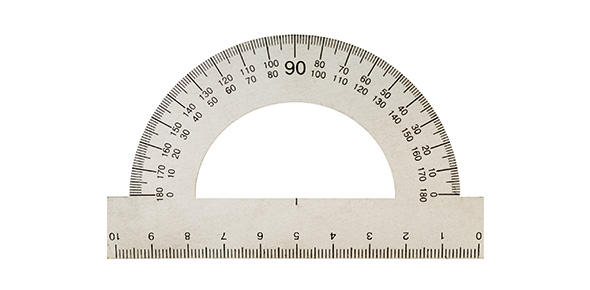# Quiz Over Metric System

18 Questions | Total Attempts: 64SettingsThis quiz is about conversions and Density. Show your work. do not write on the paper.

• 1.
Convert  0.851 L to militers/
• 2.
Convert 0.765 grams to kilograms.
• 3.
Convert 1.34 g to milligrams.
• 4.
Convert 34.2 mg to grams.
• 5.
Convert 23,745 kg to milligrams  ( Hint:  use two converstion factors)
• 6.
Convert  17.3 m to centimeters.
• 7.
Convert 2.56 m to kilometers
• 8.
Convert 5.13 m to millimeters.
• 9.
Which of the following lengths is the shortest, and which is the longest:1583 cm, 0.0128 km, 17,931 mm, and 14 m ?
• 10.
How does mass differ from weight?
• 11.
What is the mass in kilograms of a 22,000 g bag of fertilizer?
• 12.
Convert  17.3 s to milliseconds.  (Hint use two conversion factors if needed.)
• 13.
Convert 2.56 mm to kilometers.
• 14.
Convert 567 cg to grams.
• 15.
Convert 5.13 m to kilometers.
• 16.
What is the formula for density?
• 17.
What is the density  of water?
• 18.
Explain the why density of some objects float and others sink.
Related TopicsBack to top